]> 4.3.1 Example

#### 4.3.1 Example

By applying, the formulae [22, 858.652, 861.16, 861.01] we can represent the Laplace transform of the function $f\left(t\right)=\frac{sint}{t}$ ($f\in {\text{L}}^{2}\left(0,\infty \right)$) as

$\begin{array}{c}\stackrel{̂}{f}\left(x+jy\right)={\int }_{0}^{\infty }\frac{sintcosyt}{t}{e}^{-xt}dt-j{\int }_{0}^{\infty }\frac{sintsinyt}{t}{e}^{-xt}dt=\hfill \\ =\frac{1}{2}\left[arctan\left(\frac{1+y}{x}\right)+arctan\left(\frac{1-y}{x}\right)\right]+\frac{j}{4}ln\left[\frac{{x}^{2}+{\left(y-1\right)}^{2}}{{x}^{2}+{\left(y+1\right)}^{2}}\right],\phantom{\rule{1em}{0ex}}x>0,y\in \mathbb{ℝ}.\hfill \end{array}$

Now, (4.12), (4.13), (4.14), (4.16) yield the following results:

• For $n=1$, $S={\mathbb{ℝ}}^{-}$ (a real, negative ${\lambda }_{1}$) the performance index $J$,
$J\left({\lambda }_{1}\right)={\left|{〈f,{e}_{1}〉}_{{\text{L}}^{2}\left(0,\infty \right)}\right|}^{2}={\left[\sqrt{-2{\lambda }_{1}}\stackrel{̂}{f}\left(-{\lambda }_{1}\right)\right]}^{2}$

is plotted at Figure 4.2 and it has a unique local (and thus global) maximum: ${\lambda }_{1}^{\ast }=-0.7185223$, $J=1.2907889$. The corresponding approximating function takes the form

$\left[0,\infty \right)\ni t↦{〈f,{e}_{1}〉}_{{\text{L}}^{2}\left(0,\infty \right)}{e}_{1}\left(t\right)=\left[\sqrt{-2{\lambda }_{1}^{\ast }}\stackrel{̂}{f}\left(-{\lambda }_{1}^{\ast }\right)\right]\sqrt{-2{\lambda }_{1}^{\ast }}{e}^{{\lambda }_{1}^{\ast }t}.$

Its graph is compared with the graph of $f$ at Figure 4.3.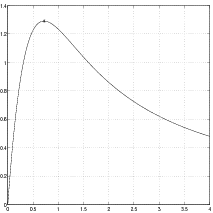Figure 4.2: Plot of the performance index. The case of one real exponent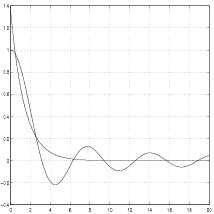Figure 4.3: Plot of the function $f$ and its approximation

• For $n=2$, $S={\mathbb{ℝ}}^{-}$ (the real and negative ${\lambda }_{1}$, ${\lambda }_{2}$) the level curves of the performance index $J$,
$J\left({\lambda }_{1},{\lambda }_{2}\right)=\sum _{k=1}^{2}{\left|{〈f,{e}_{k}〉}_{{\text{L}}^{2}\left(0,\infty \right)}\right|}^{2}$

are depicted in Figure 4.4 and $J$ has two local maxima: ${\lambda }_{1}^{\ast }={\lambda }_{2}^{\ast }=-0.40056$, $J=1.333940965$, ${\lambda }_{1}^{\ast \ast }={\lambda }_{2}^{\ast \ast }=-1.44697$, $J=1.374780723$ (the global maximum). The corresponding approximating function takes the form

$\begin{array}{c}\left[0,\infty \right)\ni t↦\sum _{k=1}^{2}{〈f,{e}_{k}〉}_{{\text{L}}^{2}\left(0,\infty \right)}{e}_{k}\left(t\right)=\hfill \\ =-2{\lambda }_{1}^{\ast \ast }\stackrel{̂}{f}\left(-{\lambda }_{1}^{\ast \ast }\right){e}^{{\lambda }_{1}^{\ast \ast }t}-2{\lambda }_{1}^{\ast \ast }\left[\stackrel{̂}{f}\left(-{\lambda }_{1}^{\ast \ast }\right)-2{\lambda }_{1}^{\ast \ast }{\stackrel{̂}{f}}^{\prime }\left(-{\lambda }_{1}^{\ast \ast }\right)\right]\left(1+2{\lambda }_{1}^{\ast \ast }t\right){e}^{{\lambda }_{1}^{\ast \ast }t}.\hfill \end{array}$

Its graph is compared with the graph of $f$ at Figure 4.5.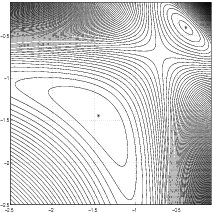Figure 4.4: Level curves of the performance index. The case of two real exponentsFigure 4.5: Plot of the function $f$ and its approximation

• For $n=2$, $S={\Pi }^{-}$ with additional requirement ${\lambda }_{1}=\overline{{\lambda }_{2}}=x+jy$, $x<0$ (clarify Remark 4.2.3) the level curves of the performance index
$J\left(x,y\right)=\sum _{k=1}^{2}{\left|{〈f,{e}_{k}〉}_{{\text{L}}^{2}\left(0,\infty \right)}\right|}^{2}$

are depicted in Figure 4.6 and $J$ has two global maxima: ${\lambda }_{1}^{\ast }=-0.39133±j0.83583={x}^{\ast }±j{y}^{\ast }$, $J=1.496017706$. The corresponding approximating function is real and takes the form

$\begin{array}{c}\left[0,\infty \right)\ni t↦{〈f,{e}_{1}〉}_{{\text{L}}^{2}\left(0,\infty \right)}{e}_{1}\left(t\right)+{〈f,{e}_{2}〉}_{{\text{L}}^{2}\left(0,\infty \right)}{e}_{2}\left(t\right)=\hfill \\ =-\frac{4{x}^{\ast }}{{y}^{\ast }}{e}^{{x}^{\ast }t}\left[Acos\left({y}^{\ast }t\right)+\frac{\left[{\left({x}^{\ast }\right)}^{2}+{\left({y}^{\ast }\right)}^{2}\right]B+A{x}^{\ast }}{{y}^{\ast }}sin\left({y}^{\ast }t\right)\right]\hfill \end{array}$

where $A:=Im\left[\left({x}^{\ast }+j{y}^{\ast }\right)\stackrel{̂}{f}\left(-{x}^{\ast }-j{y}^{\ast }\right)\right]$ and $B:=Im\left[\stackrel{̂}{f}\left(-{x}^{\ast }-j{y}^{\ast }\right)\right]$. Use the Laplace transform to conﬁrm the last formula. Its graph is compared with the graph of $f$ at Figure 4.7.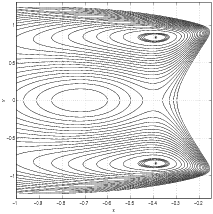Figure 4.6: Level curves of the performance index. The case of a complex conjugate pair of exponents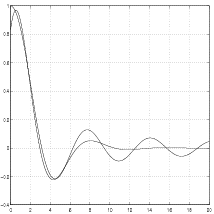Figure 4.7: Plot of the function $f$ and its approximation. The case of a complex conjugate pair of exponents

The above example shows that the numerical algorithms of global optimization should be applied to solve the problem (4.12).

Exercise 4.3.1. Repeat the above calculations for the function $f\left(t\right)=1l\left(t\right)-1l\left(t-1\right)\in {\text{L}}^{2}\left(0,\infty \right)$. Representing the Laplace transform of $f$ as

$\stackrel{̂}{f}\left(x+jy\right)=\frac{x\left(1-{e}^{-x}cosy\right)+y{e}^{-x}siny}{{x}^{2}+{y}^{2}}+j\frac{x{e}^{-x}siny-y\left(1-{e}^{-x}cosy\right)}{{x}^{2}+{y}^{2}}$

and applying formulae (4.12), (4.13), (4.14) we get:

• For $n=1$, $S={\mathbb{ℝ}}^{-}$ (a real negative ${\lambda }_{1}$) $J$ has a local maximum (which is also global) ${\lambda }_{1}^{\ast }=-1.256431209$, $J=0.8145287552$ (compare with [52, p. 403]).
• For $n=2$, $S={\mathbb{ℝ}}^{-}$ (real and negative ${\lambda }_{1}$, ${\lambda }_{2}$) $J$ has two local maxima such that ${\lambda }_{1}^{\ast \ast }={\lambda }_{2}^{\ast \ast }=-2.8427$, $J=0.886137444$ is the global maximum.
• For $n=2$, $S={\Pi }^{-}$ with the requirement that ${\lambda }_{2}={\overline{\lambda }}_{1}$ $J$ has two global maxima: ${\lambda }_{1}^{\ast }=-1.511±j1.82618$, $J=0.9139904969$.